Next: Implementation of a reservoir Up: Wave propagation in an Previous: Introduction

# Reservoir fluid flow simulatorUnfortunately, a reservoir is difficult to access. It is buried hundreds or thousands of meters in the subsurface. A geoscientist only chance to access the reservoir directly is in a well. Petroleum engineers carefully observe a well's production volumes of oil, water, and gas. Additionally, the engineers measure pressure in the well or even conduct pressure tests. My discussion of reservoir fluid flow processes follows Landa 1997 and Aziz 1979.

Black-oil model. Petroleum engineers use various reservoir fluid flow simulators to relate their observations to reservoir properties, such as permeability, porosity, pressure distribution, and saturation. The standard black-oil model for a reservoirs mix of fluids comprises three distinct phases: oil, water, and gas. The oil phase dissolves a certain amount of gas; the water does not. Intuitively, one might want to describe the reservoir properties at the scales of pores. However, the traditional approach homogenizes the pore-scale heterogenities to macroscopic descriptions such as Darcy's law.

The general equation of mass conservation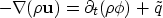(1)
asserts that any local change in the flow of mass is due to a local source (or sink)or a temporal change in density or porosity. The sourceis a location's local well production which is zero for any location without a producing well. The vectoris the fluid flux, andis the fluid density.

Darcy's law expresses the fluid flux in terms of pressure and gravity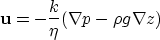(2)
where k is the permeability, andis the viscosity. The second term on the right hand side incorporates the gravitational effect, where g is the gravitational acceleration. Darcy's law is only valid for slow flow rates, where turbulence and inertia are negligible. Darcy's law 2 generalizes to the flow of multiple phases - oil, water, gas - in a porous rock: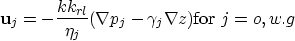where krl is the relative permeability of each phase. The relative permeability is a function of saturation. Aziz 1979 discusses various empirical expressions for that dependency.

The combination of Darcy's law with the mass conservation equation 1 yields the fluid flow equations:(3)
The equations relate differences in pressure gradient - left hand side - to temporal changes in pore saturation - right hand side. The equations are complemented by three additional constraints(4)
The first equation states that the rock is saturated by the three fluids. The two bottom equations relate capillary pressures and saturations and are usually empiric Aziz and Settari (1979).

The parameters Bj relate parameter values at surface conditions - indicated by index S - and reservoir condition - indicated by index R. The parameters are defined as volume ratio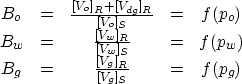(5)
and are based on the pressure-volume-temperature (PVT) constant at thermodynamic equilibrium. The first equation deviates from the other two since it accounts for the dissolved gas volume Vdg. The volume ratios Bj let us relate the density at reservoir conditions to the density at surface conditions: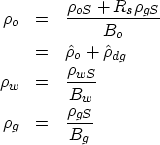(6)
The mass transfer between the oil and gas phase is defined as Rs = [Vdg / Vo]S.

The Bj ratios, and the mass transfer Rs, depend on the pressure pj of the various phases j=o,w,g. Since water compressibility is small, the relationship Bw = f(pw) can be approximated byThe analogue expressions for Bo, Bg, Rs, andare empiric and again discussed by Aziz and Settari (1979).

The coefficients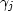in equation 4 express transmissibilities and are defined asViscositiesof oil and gas strongly depend on temperature and pressure. The pressure dependency is again empirical Aziz and Settari (1979). The viscosity's temperature dependence is especially important in thermal-recovery processes such as steam injections. In general, the temperature dependency is well approximated by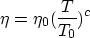where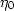and T0 are reference points and c is a fluid specific constant.

Finally, porosity is often pressure dependentwhere cR is the bulk compressibility of the rock and the porosity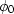and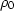refer to known reference values.

The equations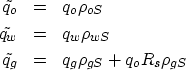(7)
relate the in situ volumesof equation 1 to the production volumes q as they appear at the well head under standard surface condition.

Well observations. In a field experiment, petroleum engineers control the pressure in the well. Petroleum engineers conduct well tests by changing the pressure in the well and observing the reaction of the surrounding reservoir pressure (respectively its effect on the controlled well pressure). These experiments attempt to estimate local reservoir transmissibility but do have to account for the skin effect: the alterations of the rock next to the well. Additionally, petroleum engineers record the well's production volumes. The production volumes depend on the reservoir pressure p and the well bore pressure pwf: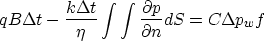where q the volume production rate per unit of time at the surface (not at reservoir pressure), B is the formation volume factor, C represents the ability of the well bore to store fluids, n is the normal of the well bore, S the well bore's surface.

I hope to combine such well observations with seismic time-lapse data to yield improved estimates of reservoir property maps.

Simplified well system My preliminary implementation is based on the assumption that the reservoir only contains a single fluid, e.g. oil but neither water, nor gas. This assumption might be locally reasonable for large oil fields, albeit I expect almost all hydrocarbon mixtures to release gas when a production lowers the reservoir pressure sufficiently. The assumption of a single fluid phase collapses the fluid flow equations 4 and its constraints 4 to a single equation.

Additionally, I neglect gravitational forces (g =0). I assume that the rocks transmissibility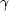varies smoothly and that the rocks pore volume is independent of pressure (cR = 0). Finally, I suggest that the reservoir fluid is only slightly compressible and consequently satisfiesUnder these assumption the equation system for multiple phases collapses to the diffusivity equation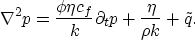(8)
This single phase fluid flow equation for porous rocks can be derived directly from the corresponding versions of Darcy's law and the mass conservation Raghavan (1993).

I doubt that seismic field data can detect reasonable changes in pressure and density of a single fluid phase around a well. Instead, current time lapse surveys concentrate on detecting saturation changes in multi-phase reservoirs, that often involve gas, steam, or highly pressured water. I chose the single fluid phase case since it is the simplest to implement. Should I continue this work, I will seek the collaboration of an experienced petroleum engineer to improve the reservoir flow simulator. The simplicity of the reservoir model, however, might prove beneficial when formulating the inverse problem.

Parameterization My simple reservoir simulator requires as input a transmissibility map of the reservoir, an initial pressure distribution within the reservoir (usually assumed to be constant), the pressure history at the boundary of the wells. Given these parameters, the simulator computes the reservoir pressure distribution over time. For this preliminary study, I restrict the reservoir to a two-dimensional plane. The model is computationally manageable, and the results can be visualized effectively.Next: Implementation of a reservoir Up: Wave propagation in an Previous: Introduction
Stanford Exploration Project
3/9/1999